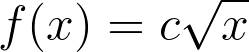Day 17 - Derivative Rules - 09.09.15

Update
• Athlete of the Month
• Block 1
• In order to collect information to better serve BHS students, the Counseling and Advising Department wants you to complete the All School Survey 2015. So please listen carefully to the following directions. If you have problems logging in, please try again at a later time as we may have connectivity issues. The survey should take about five minutes to administer and complete.
2. Go to the BHS homepage. Click on the STUDENTS tab on the top of the page.
3. A drop down menu will appear, click on the Naviance link found at the bottom of the middle column. You will be redirected to the Naviance page. It will have a red background.
6. At this point you should be in your Naviance account.
7. Click on the “About Me” tab.
8. On the left side of the page you will see a heading titled: Surveys to Take.
9. Under the Surveys to take heading, click on the survey titled: All School Survey 2015.
11. Click the update button at the end.

Bell Ringer

1. What is the derivative of the following: f(x) = c

1. f’(x) = c

2. f’(x) = 1

3. f’(x) = 0

4. does not exist

5. none of the above

2. What is the derivative of the following: f(x) = cx

1. f’(x) = c

2. f’(x) = 1

3. f’(x) = 0

4. does not exist

5. none of the above

3. What is the derivative of the following: f(x) = cx2

1. f’(x) = 2cx

2. f’(x) = 2c

3. f’(x) = c

4. f’(x) = 0

5. none of the above

4. What is the derivative of the following:1.2.3.4. does not exist

5. none of the above

5. What is the derivative of the following: f(x) = x2 + x + 1

1. f’(x) = 2x

2. f’(x) = 2x + 1

3. f’(x) = x + 1

4. does not exist

5. none of the above

Review

Lesson

Exit Ticket
• Posted on the board at the end of the block.

Homework
• WNQ the following:

Lesson Objectives
• How can basic derivatives rules be calculated?

In-Class Help Requests

Standard(s)
• APC.5
• Investigate derivatives presented in graphic, numerical, and analytic contexts and the relationship between continuity and differentiability.
• The derivative will be defined as the limit of the difference quotient and interpreted as an instantaneous rate of change.
• APC.6
• ​The student will investigate the derivative at a point on a curve.
• Includes:
• finding the slope of a curve at a point, including points at which the tangent is vertical and points at which there are no tangents
• using local linear approximation to find the slope of a tangent line to a curve at the point
• ​defining instantaneous rate of change as the limit of average rate of change
• approximating rate of change from graphs and tables of values.